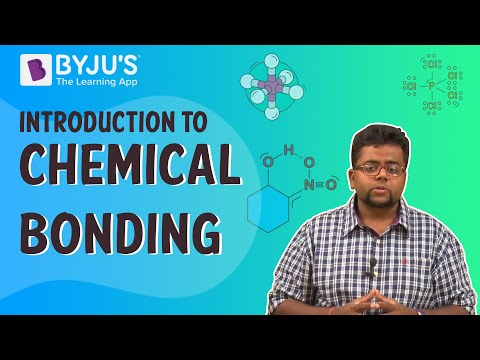Checkout JEE MAINS 2022 Question Paper Analysis : Checkout JEE MAINS 2022 Question Paper Analysis :

# Chemical Equation Questions

Chemists use chemical equations to describe chemical reactions, which transform one form of matter (reactants) into another form of matter (products). The unbalanced chemical reaction of methane (CH4) combustion with oxygen (reactants) to produce carbon dioxide and water is shown below (the products).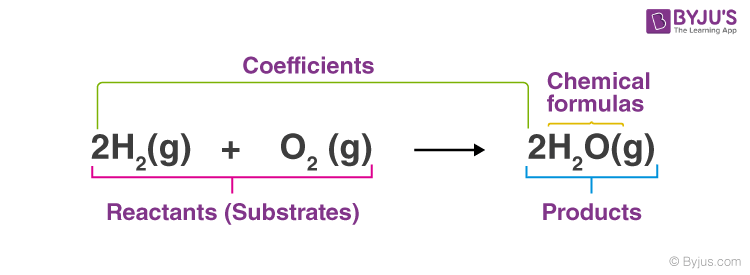Definition: Chemical equations are symbolic expressions of chemical reactions that express the reactants and products in terms of their chemical formulae. Chemical equations also use symbols to depict factors such as the direction of the reaction and the physical states of the reacting entities. In 1615, the French chemist Jean Beguin was the first to establish chemical equations.

## Chemical Equation Chemistry Questions with Solutions

Q1: What is an Ionic Equation?

Ionic equations are chemical equations where electrolytes are depicted as dissociated ions. They are frequently employed to illustrate the displacement reactions in aqueous media. Some ions participate in these processes, whereas others are not. Spectator ions do not react and are generally left out of the overall ionic equation.

Q2: What is Balanced Chemical Equation?

A balanced equation is a chemical reaction in which the overall charge and the number of atoms for every element in the reaction are the same for both the reactants and the products. In other respect, the mass and charge on either side of the reaction are equal.

Chemical equations for mass and charge are usually balanced in aqueous solutions. On both sides of the equation, balancing for mass yields the same number and types of atoms. When the net charge on both sides of the equation is zero, the equation is said to be balanced for the charge.

Q3: “We need to balance a skeletal chemical equation.” Give a reason to justify the statement.

Skeletal chemical equations are usually unbalanced. Because of the law of conservation of mass, we must balance the chemical equation. ‘Matter can neither be generated nor destroyed,’ it says. As a result, every chemical reaction must have a balanced chemical equation.

Q4: Why does a wall not immediately acquire a white colour when a coating of slaked lime is applied?

Slaked lime is not particularly white. CO2 gas in the air reacts with calcium hydroxide to generate calcium carbonate. It’s fairly white, so it gives the wall a white appearance.

Ca(OH)2 + CO2(g) → CaCO3(s) + H2O(l).

Q5: A student burnt a metal A found in the form of ribbon. The ribbon burnt with a dazzling Flame & a white powder B is formed, which is basic. Identify A & B. Write the Balanced chemical equation.

A = Mg, B = MgO. 2Mg + O2 → 2MgO

Q6: A balanced chemical equation is in accordance with-

(i) Multiple proportion

(ii) Reciprocal proportion

(iii) Conservation of mass

(iv) Definite proportions

Answer: (iii) Law of Conservation of Mass

Q7: Magnesium ribbon is rubbed before burning because it has a coating of

(i) basic magnesium oxide

(ii) basic magnesium carbonate

(iii) basic magnesium sulphide

(iv) basic magnesium chloride

Q8: Identify the substance oxidised in the below equation.

MnO2 + 4HCl → MnCl2 + 2H2O + Cl2

(i) MnCl2

(ii) HCl

(iii) MnO2

(iv) H2O

Explanation: In this reaction HCl is oxidised to Cl2, whereas MnO2 is reduced to MnCl2.

Q9: A researcher adds barium hydroxide to hydrochloric acid to form a white-coloured barium chloride. Which option gives the balanced chemical equation of the reaction?

(i) HCl + Ba(OH)2 → BaCl2 + 2HOH

(ii) 2HCl + Ba(OH)2 → BaCl2 + 2HOH

(iii) 2HCl + Ba(OH)2 → BaH2 + 2HCl + O2

(iv) HCl + 2Ba(OH) → 2BaCl2 + 2HOH + O2

Answer: (ii) 2HCl + Ba(OH)2 → BaCl2 + 2HOH

Q10: In the double displacement reaction between aqueous potassium iodide and aqueous lead nitrate, a yellow precipitate of lead iodide is formed. While performing the activity, if lead nitrate is not available, which of the following can be used instead of lead nitrate?

(ii) Potassium sulphate

(iii) Ammonium nitrate

Q11: When SO2 gas is passed through saturated solution of H2S, which of the following reaction occurs?

(i) SO2 + 2H2S → 2H2O + 3S

(ii) SO2 + 2H2S → H2O + 3S

(iii)SO2 + H2S → H2O + S

(iv) SO2 + H2O → SO3 + H2

Answer: (i) SO2 + 2H2S → 2H2O + 3S

Q12: With the help of an activity show that iron is more reactive than copper?

To begin, dip iron nails into an aqueous solution of CuSO4 (blue). Leave for half an hour. The solution’s blue colour fades to light green. Brown deposits occur on the nails at the same time. Because iron is in the reactivity series above copper, it displaces copper from the CuSO4 solution. Copper is present in the brown deposit. The chemical equation is given below:

Fe(s) + CuSO4(aq) → FeSO4(aq) + Cu(s)

Q13: State the type of chemical reactions and chemical equations that take place in the following:

(i) Magnesium wire is burnt in air.

(ii) Electric current is passed through water.

(iii) Ammonia and hydrogen chloride gases are mixed.

(i)

$$\begin{array}{l}2Mg(s) + O_{2}(g) \to 2MgO(s)\end{array}$$

Combination reaction (Redox reaction).

(ii)

$$\begin{array}{l}2H_{2}O(l) \xrightarrow[]{Electrolysis} 2H_{2}(g) + O_{2}(g)\end{array}$$

Electrical decomposition reaction.

(iii)

$$\begin{array}{l}NH_{3}(g) + HCl(g) \to NH_{4}Cl(s)\end{array}$$

Combination reaction.

Q14: (i) Write the essential condition for the following reaction:

2AgBr → 2Ag + Br2.

Write one application of this reaction.

(ii) Complete the following chemical equation of a chemical reaction 2FeSO4

$$\begin{array}{l}2FeSO_{4} \overset{Heat}{\rightarrow} Fe_{2}O_{3} + … + …\end{array}$$

(iii) What happens when water is added to quicklime? Write the chemical equation.

(i)

$$\begin{array}{l}2AgBr \xrightarrow[]{Sunlight} 2Ag + Br_{2}\end{array}$$

In photography, this reaction is used.

(ii)

$$\begin{array}{l}2FeSO_{4} \overset{Heat}{\rightarrow} Fe_{2}O_{3} + SO_{2} + SO_{3}\end{array}$$

(iii) With a hissing sound and a lot of heat, slaked lime is produced.

$$\begin{array}{l}CaO + H_{2}O \to Ca(OH)_{2}\end{array}$$

Q15: (a) Explain two ways by which food industries prevent rancidity.

(b) Discuss the importance of decomposition reaction in the metal industry with three points.

(a) (i) Antioxidants can be added to foods containing fat and oil to avoid rancidity. For example, butylated hydroxyanisole is added to butter as an antioxidant.

(ii) It can also be prevented by packing foods high in fat and oil in nitrogen gas.

(b) (i) Sodium metal is formed when molten NaCl is electrolytically dissolved.

(ii) Electric decomposition of bauxite ore coupled with cryolite produces aluminium metal.

(iii) Thermal decomposition of carbonate ores produces metal oxide, which is reduced to metal.

## Practise Questions on Chemical Equation

Q1: How to balance chemical equations?

Q2: Identify the type of chemical reaction:

(i) A → B + C

(ii) AB + CD → AD + CB

Q3: What is observed when a solution of potassium iodide solution is added to a solution of lead nitrate? Name the type of reaction. Write a balanced chemical equation to represent the above chemical reaction.

Q4: Aluminium is a reactive metal but is still used for packing food articles. Why?

Q5: (a) Why is combustion reaction an oxidation reaction?

(b) How will you test whether the gas evolved in a reaction is hydrogen?

(c) Why does not silver evolve hydrogen on reacting with dilute sulphuric acid?

Click the PDF to check the answers for Practice Questions.

## Recommended Videos

#### NCERT Grade 10 Chemistry Chemical Reactions and Equations Balancing of a Chemical Reaction Q04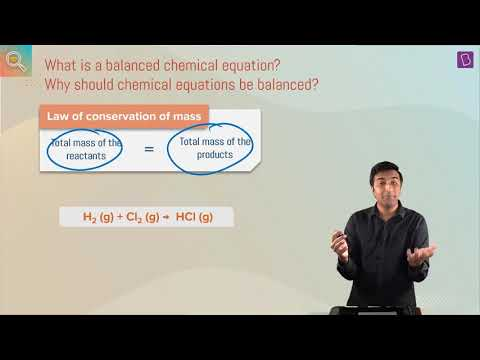#### Balancing A Chemical Equation I Class 10 I Learn With BYJU’S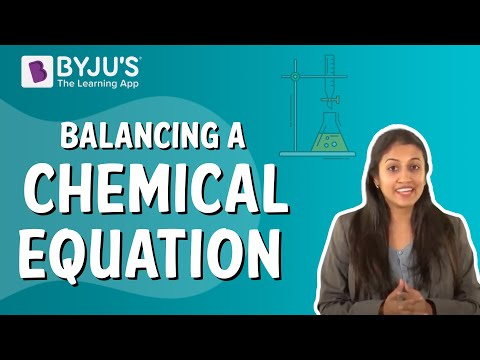#### NCERT Grade 10 Chemistry Chemical Reactions and Equations Balancing of a Chemical Reaction Q06.4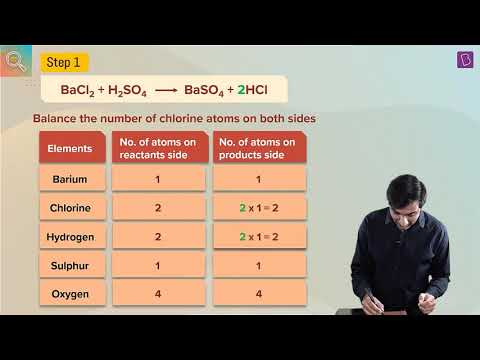#### Introduction to Chemical Bonding